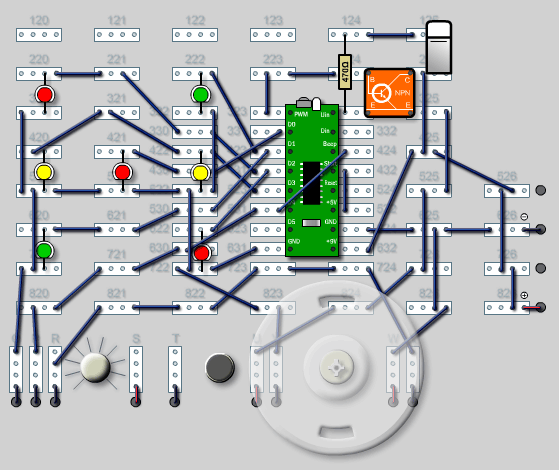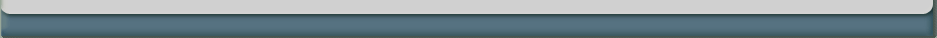Experiments for the Thames and Kosmos Computer Systems Engineering KitProgram cp_dice.k

Rem Dice
Pdir = 63
L1: Random Portout = 1
Gosub L4: Input Check
Portout = 2
Gosub L4: Input Check
Portout = 3
Gosub L4: Input Check
Portout = 6
Gosub L4: Input Check
Portout = 7
Gosub L4: Input Check
Portout = 14
Gosub L4: Input Check
Goto L1: Random
L2: Dice down D = 1
PWM = 0
Beep
Delay = 255
L3: Dice falling A = D
B = 0
If A = B Goto L2: Dice down
L4: Input Check A = Uin
PWM = 0
Delay = 3
B = 6
If A < B Goto L3: Dice falling
D = 0
PWM = A
L5: SoundPortout = 16
Portout = 0
Delay = 30
Return
End